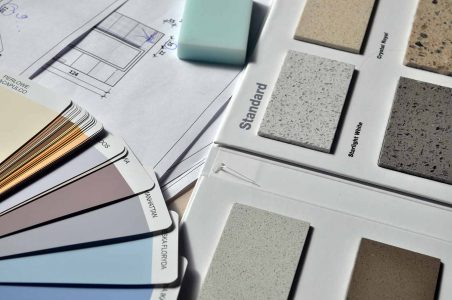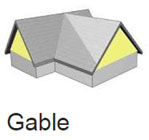Exterior cladding, or siding as it is often called, can change the entire look of a house. It can give it an entirely different look and feel as compared to the inside. That is, if you are going for that contrast. Otherwise it can complement the interiors of your home as well. Hence it is a significant part of your home’s curb appeal. Measuring accurately and ordering correctly are essential to a successful siding project. Determining your siding correctly saves you money and time by eliminating the need for multiple trips to and from the supplier. It requires no special tools and only minimal mechanical and mathematical skills.

Measuring the total square footage is essential in receiving a proper cost estimate from the contractor or if you are installing the project yourself, it gives you an exact idea of how much material needs to be bought. Cost per square foot is a main factor when determining overall cost by a contractor. Also, as online DIY groups suggest: Don’t overlook the complexity of the house structure, a single story small home could cost just as much as a large 2 story home, if there are very complex angles and variance on the exterior siding. The more windows, doors, & complex variance in the structure the more measuring & cutting driving up labour & material costs.Actual siding costs will include the following prices:
– The cost of the siding material as well as the transportation to the residential home
– Cost of manual labour for removal of existing siding
– Cost of labour as well as preparation of the house for installation
– Installation charges of the siding, finishing, & clean up### Procedure

1. Measure the height of the house from the top of the foundation to the top (W). Measure around the perimeter of the building (P). Use our calculator to multiply P by W to get square footage (SF). These lines should be measured in feet (ft) for square footage calculations and if needed, converted to inches (in), yards (yd), centimetres (cm), millimetres (mm) and metres (m). To convert among square feet, square inches, square yards, square centimetres, square millimetres and square meters you can utilize the conversion table located at the bottom of our calculators. Our tools give you the option of calculating the exact cost of materials. All you have to do is enter the price per unit area and voila, you have the total cost of materials in a single click!

Area of A Square/Rectangle

#### Formula:

W x P = Square Footage
Abbreviations of unit area: ft2, in2, yd2, cm2, mm2, m2

2. Measure the height (H) and length (L) of each gable. A gable is the generally triangular portion of a wall between the edges of intersecting roof pitches. Multiply H and L by 1/2 to get gable square footage (GSF). Add GSF to SF from Step 1 to get total square footage (TSQ).Area of a Triangle

#### Formula:

(H X L)/2 = GSF
GSF + SF = TSQ
Abbreviations of unit area: ft2, in2, yd2, cm2, mm2, m2

You might need a combination of the below calculators to figure out the exact area of the structure you are trying to cover:

Area of a Circle

The area of a circle is the space contained within its circumference (outer perimeter). To find out the area of a circle, we need to know its diameter which is the length of its widest part. The diameter should be measured in feet (ft) for square footage calculations and if needed, converted to inches (in), yards (yd), centimetres (cm), millimetres (mm) and metres (m).

#### Formula:

Area of a Circle = π x (Diameter/2)2
π = 3.142
Answer = (π x (Diameter/2)2) square area
Abbreviations of unit area: ft2, in2, yd2, cm2, mm2, m2

Area of a Trapezoid

To find out the area of a trapezoid, we need to know the length of two parallel sides and the distance (height) between them. The sides should be measured in feet (ft) for square footage calculations and if needed, converted to inches (in), yards (yd), centimetres (cm), millimetres (mm) and metres (m).

#### Formula:

Area of a Trapezoid = ((a + b) / 2) h
Answer = (((a + b) / 2) h) square area
Abbreviations of unit area: ft2, in2, yd2, cm2, mm2, m2

3. Divide TSQ by 100 to determine the number of squares need (SN). A square is a 10-foot by 10-foot area, or 100 square feet. Order SN squares of siding.

Siding Square Footage Calculator

#### Formula:

TSQ/100 = SN

So now you know how to calculate siding square footage for a structure. Write to us if you need us to cover more of such topics. Please note that the above calculator is just an online estimation tool, and should not be taken as a firm price.